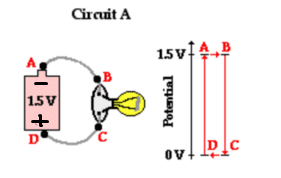# How do i interpret the following electric potential diagram?

• WhyNerfZed

#### WhyNerfZed

We recently started to learn about electricity in school and I'm trying to understand the concept of voltage(potential differance). From what i understand, electrons flow from the negative terminal to the positive. The electrons at the negative terminal will have 1.5 J of potential energy per Columb(see diagram), and when they reach the positive terminal, all the potential energy will have been transformed.1. The diagram to the right shows how the voltage drops in the circuit. According to the illustration, the electrical potential remains constant from point A to point B. But is this the case? Isn't electric potential really energy due to position per columb? And since the position change, shouldn't the potential change aswell? Similary, after the charges pass the lightbulb the diagram shows that all the potential energy has been transformed to light and thermal energy. But wouldn't there be some potential energy left, since a charge at position C has potential energy with respect to the positive terminal.

2. If the only cause of voltage drop in the circuit is when the charges encounters the lighbuld, then that suggests there would be no drop in potential from A to B if the circuit didn't have the lightbulb. This doesn't make sense to me.

3. Is the potential energy of a negatively charged particle in the negative terminal equal to the work needed to "push" it from the positive terminal to the negative terminal in the internal circuit, in other words is it equal to E * Q * d, where E is the electric field strength, Q is the charge of the particle and d is the distance between the terminals.
I would greatly appreciate it if you can answer/correct any or all of these questions.

Last edited:

The potential at the positive terminsl is 1.5V bigger than the potential at the negative terminal... so the potential difference is 1.5V or -1.5V depending on which way around you do the subtraction.

When a positive charge goes from the negative terminal to the positive one, it gains 1.5V, going the other way, it loses 1.5V.

Answer to all questions in 1 are "no".
The potential difference is like a difference in height... a ball rolls down a hill, but, once it got going, rolls along flat ground without changing potentual.

2. Thats a flaw in the model where the wires are ideal conductors. This does not happen irl.

Isn't electric potential really energy due to position per columb? And since the position change, shouldn't the potential change aswell?
I think this is the nub of your problem. 'Potential' refers to a particular place in the circuit (and also Potential Difference), while 'Potential Energy' refers to the energy of a distinct set of charges. So the PE of a quantity of charge will change as it moves round a circuit but the PD will stay the same.
It's the same with gPE of an object above ground (mgh) and gP, the potential of its position (gh).
Q2. using the gravitational analogy, a perfect wire is equivalent to a perfectly horizontal shelf. As a ball rolls across the shelf, its gPE doesn't change and no energy is involved or lost as it enters at one end of the shelf and leaves at the other end. The ball's KE is the same at the start and at the end . In practice, a miniscule amount of energy is dissipated as charges flow through real wires but the wires, drawn on a diagram have no resistance at all.